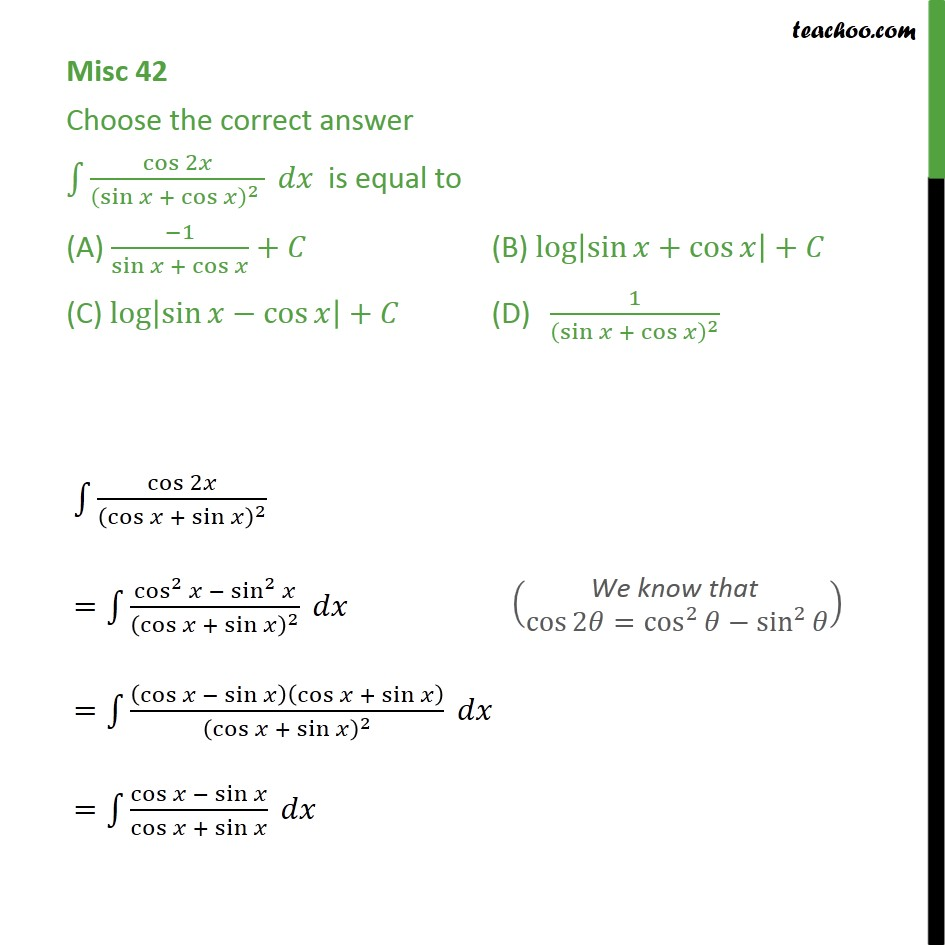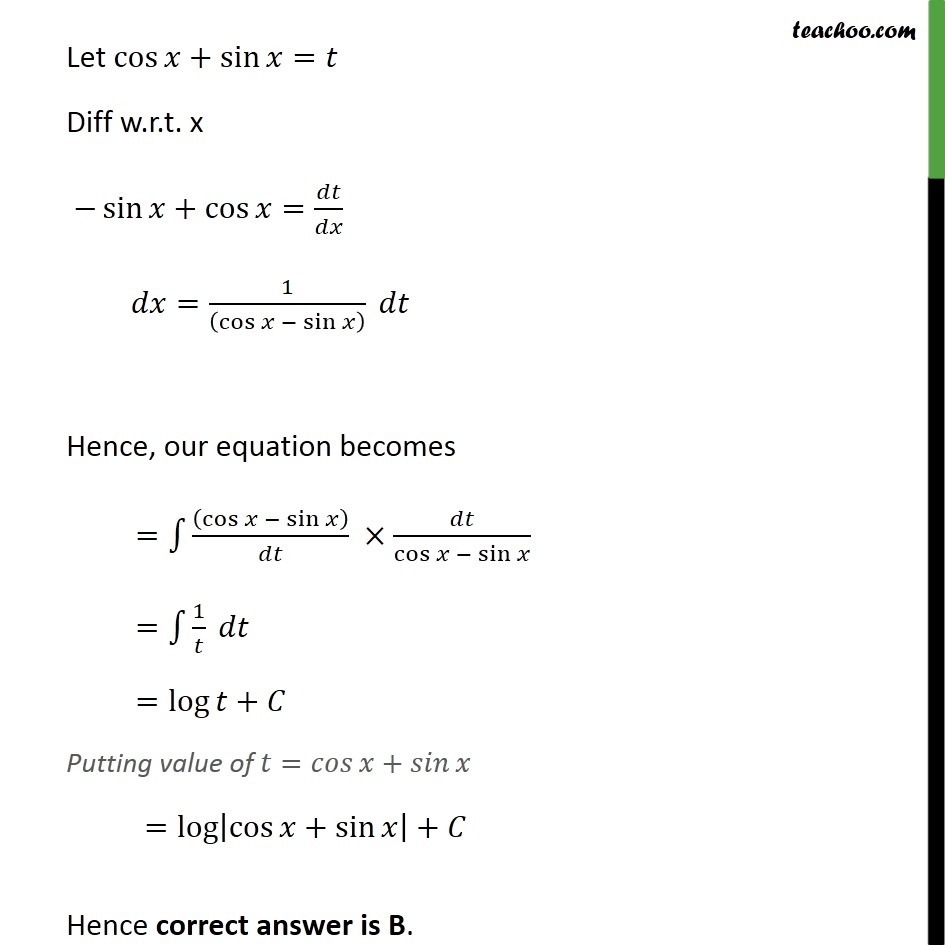Miscellaneous

Chapter 7 Class 12 Integrals (Term 2)
Serial order wise### Transcript

Misc 42 Choose the correct answer ﷐﷮﷮﷐﷐cos﷮2𝑥﷯﷮﷐﷐﷐sin﷮𝑥﷯ + ﷐cos﷮𝑥﷯﷯﷮2﷯ ﷯﷯ 𝑑𝑥 is equal to (A) ﷐−1﷮﷐sin﷮𝑥﷯ + ﷐cos﷮𝑥﷯﷯+𝐶 (B) ﷐log﷮﷐﷐sin﷮𝑥﷯+﷐cos﷮𝑥﷯﷯﷯+𝐶 (C) ﷐log﷮﷐﷐sin﷮𝑥﷯−﷐cos﷮𝑥﷯﷯﷯+𝐶 (D) ﷐1﷮﷐﷐﷐sin﷮𝑥﷯ + ﷐cos﷮𝑥﷯﷯﷮2﷯﷯ ﷐﷮﷮﷐﷐cos﷮2𝑥﷯﷮﷐﷐﷐cos﷮𝑥﷯ + ﷐sin﷮𝑥﷯﷯﷮2﷯﷯﷯ =﷐﷮﷮﷐﷐﷐cos﷮2﷯﷮𝑥﷯ − ﷐﷐sin﷮2﷯﷮𝑥﷯﷮﷐﷐﷐cos﷮𝑥﷯ + ﷐sin﷮𝑥﷯﷯﷮2﷯﷯﷯ 𝑑𝑥 =﷐﷮﷮﷐﷐﷐cos﷮𝑥﷯ − ﷐sin﷮𝑥﷯﷯﷐﷐cos﷮𝑥﷯ + ﷐sin﷮𝑥﷯﷯﷮﷐﷐﷐cos﷮𝑥﷯ + ﷐sin﷮𝑥﷯﷯﷮2﷯﷯﷯ 𝑑𝑥 =﷐﷮﷮﷐﷐cos﷮𝑥﷯ − ﷐sin﷮𝑥﷯﷮﷐cos﷮𝑥﷯ + ﷐sin﷮𝑥﷯﷯﷯ 𝑑𝑥 Let ﷐cos﷮𝑥﷯+﷐sin﷮𝑥﷯=𝑡 Diff w.r.t. x −﷐sin﷮𝑥﷯+﷐cos﷮𝑥﷯=﷐𝑑𝑡﷮𝑑𝑥﷯ 𝑑𝑥=﷐1﷮﷐﷐cos﷮𝑥﷯ − ﷐sin﷮𝑥﷯﷯﷯ 𝑑𝑡 Hence, our equation becomes =﷐﷮﷮﷐﷐﷐cos﷮𝑥﷯ − ﷐sin﷮𝑥﷯﷯﷮𝑑𝑡﷯﷯ ×﷐𝑑𝑡﷮﷐cos﷮𝑥﷯ − ﷐sin﷮𝑥﷯﷯ =﷐﷮﷮﷐1﷮𝑡﷯﷯ 𝑑𝑡 =﷐log﷮𝑡﷯+𝐶 Putting value of 𝑡=𝑐𝑜𝑠⁡𝑥+𝑠𝑖𝑛⁡𝑥 =﷐log﷮﷐﷐cos﷮𝑥﷯+﷐sin﷮𝑥﷯﷯﷯+𝐶 Hence correct answer is B.